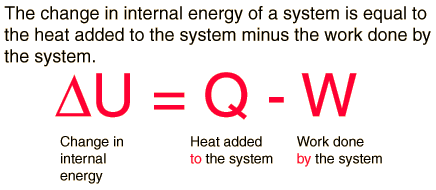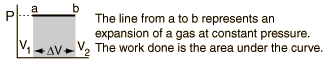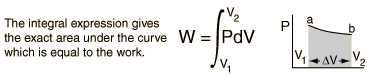# 1st law of Thermodynamics

First Law of Thermodynamics

1. Thermodynamics

Thermodynamics is a branch of natural science concerned with the interrelationship and inter-conversion of different forms of energy and the behavior of macroscopic systems in terms of certain basic quantities (such as temperature, internal energy, entropy, and pressure) that characterize materials and radiation, and explains how they are related.

• 1st Law:

The first law of thermodynamics is the application of the conservation of energy principle to heat and thermodynamic processes, and makes use of the key concepts of internal energy, heat, and system work. It is used extensively in the discussion of heat engines. The standard unit for all these quantities would be the joule, although they are sometimes expressed in calories or BTU. It is often formulated by stating that:The increase in internal energy of a closed system for any process of interest between an initial and a final state of internal thermodynamic equilibrium is equal to the change in internal energy for a reference process consisting only of adiabatic work that goes from that initial to that final state. It is also often formulated by stating that when a closed system has a change of state, and its internal energy is changed only by work and not by heat transfer, then the net amount of work transferred is the same for all arrangements of work transfer that are possible for that change of state. Also, when two systems, open to each other for transfer of matter and energy, interact but are otherwise isolated, then the sum of their internal energies does not change.

If a system has a definite temperature, then its total energy has three distinguishable components. If it is in motion, it has kinetic energy. If it is in an externally imposed force field (e.g. gravity), it has potential energy. And it has internal energy which is the sum of the kinetic energy of microscopic motions of its constituent atoms, and of the potential energy of interactions between them. Other things being equal, the kinetic energy of microscopic motions of the constituent atoms increases as the system's temperature increases. The establishment of the concept of internal energy is the characteristic distinguishing feature of the first law of thermodynamics.

• Enthalpy

Four quantities called "thermodynamic potentials" are useful in the chemical thermodynamics of reactions and non-cyclic processes. They are internal energy, the enthalpy, the Helmholtz free energy and the Gibbs free energy. Enthalpy is defined by

H = U + PV

[Enthalpy = Internal Energy + (Pressure x Volume)]

Enthalpy is then a precisely measurable state variable, since it is defined in terms of three other precisely definable state variables. It is somewhat parallel to the first law of thermodynamics for a constant pressure system

Q = ΔU + PΔV (since in this case Q=ΔH)

It is a useful quantity for tracking chemical reactions. If as a result of an exothermic reaction some energy is released to a system, it has to show up in some measurable form in terms of the state variables. An increase in the enthalpy H = U + PV might be associated with an increase in internal energy which could be measured by calorimetry, or with work done by the system, or a combination of the two. The internal energy U might be thought of as the energy required to create a system in the absence of changes in temperature or volume. But if the process changes the volume, as in a chemical reaction which produces a gaseous product, then work must be done to produce the change in volume. For a constant pressure process the work you must do to produce a volume change ΔV is PΔV. Then the term PV can be interpreted as the work you must do to "create room" for the system if you presume it started at zero volume.

• System Work

When work is done by a thermodynamic system, it is ususlly a gas that is doing the work. The work done by a gas at constant pressure is:For non-constant pressure, the work can be visualized as the area under the pressure-volume curve which represents the process taking place. The more general expression for work done is:Work done by a system decreases the internal energy of the system, as indicated in the First Law of Thermodynamics. System work is a major focus in the discussion of heat engines.For example, when a machine lifts a heavy object upwards, some energy is transferred from the machine to the object. The object acquires its energy in the form of gravitational potential energy in this example.

References: